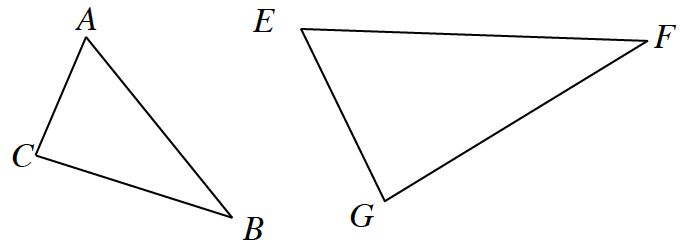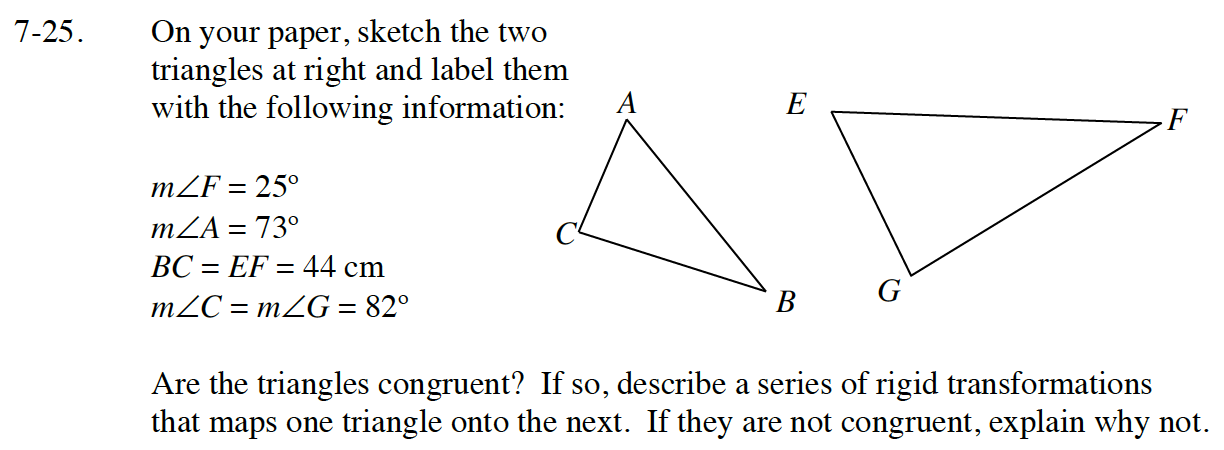Home > CC4 > Chapter 7 > Lesson 7.1.2 > Problem7-25

7-25.
1.On your paper, sketch the two triangles at right and label them with the following information: Homework Help ✎

2. mF = 25º
mA = 73º
BC = EF = 44 cm
mC = mG = 82º

3. Are the triangles congruent? If so, describe a series of rigid transformations that maps one triangle onto the next. If they are not congruent, explain why not.The sketch has been done for you.
Remember that the interior angles of a triangle total 180°.
Set up an equation for each triangle to help find the measure of the unknown angles.
How does this help you to determine if the triangles are congruent?

Remember congruent means that all corresponding angles are equal and all corresponding side are the same measure.